• 计算下面数学表达式的值：cos3.56+log1035+ln8.56+e2.63+tan0.78 输入：无。 输出：表达式的值。
• 中缀转后缀 需要建一个操作符栈op和一个字符数组expop栈存放操作符字符数组用来存放转换以后的后缀表达式首先得到用户输入的中缀表达式将其存入str数组中对str数组逐个扫描如果是数字或小数点则直
• 问题:用户输入一个包含“+”、“-”、“*”、“/”、正整数和圆括号的合法数学表达式，计算该表达式的运算结果 C语言 我这里是使用两个栈实现的，直接对中缀表达式进行运算的 输入的算式以#结尾 除此之外该问题...
问题:用户输入一个包含“+”、“-”、“*”、“/”、正整数和圆括号的合法数学表达式，计算该表达式的运算结果
C语言
我这里是使用两个栈实现的，直接对中缀表达式进行运算的
输入的算式以#结尾
除此之外该问题还可以使用一个栈，如:先转化成后缀表达式；或者使用char型共享栈，操作数进出栈时进行类型转换即可。
Evaluation.h
#ifndef EVALUATION_H_INCLUDED
#define EVALUATION_H_INCLUDED

#include <stdio.h>
#include <stdlib.h>
#define MAXSIZE 20
//操作数
typedef struct Operand
{
int Data[MAXSIZE];
int top;
}Rand;
//运算符
typedef struct Operator
{
char Data[MAXSIZE];
int top;
}Rator;
//定义栈存放操作数和运算符
Rand operands;
Rator operators;
//初始化栈
void InitOperand(Rand *ra);
void InitOperator(Rator *op);
//判栈空
int EmptyRand(Rand *ra);
int EmptyRator(Rator *op);
//进栈
int PushRand(Rand *ra,int e);
int PushRator(Rator *op,char e);
//出栈
int PopRand(Rand *ra,int *e);
int PopRator(Rator *op,char *e);
//取栈顶元素
int GetTopRand(Rand *ra);
char GetTopRator(Rator *op);
//判断字符是否为运算符
int InOp(char ch);
//判断运算符优先级
int Priority(char s);
//比较运算符优先级
int Precede(char op1,char op2);
//判断符号并运算
int Calculation(int a,int b,char c);
//计算表达式
int ExpCalculation(Rand *ra,Rator *op);
#endif // EVALUATION_H_INCLUDED

Evaluation.c
#include "Evaluation.h"
//初始化栈
void InitOperand(Rand *ra)
{
ra->top = -1;
}
void InitOperator(Rator *op)
{
op->top = -1;
}
//栈栈空
int EmptyRand(Rand *ra)
{
ra->top = -1;
return 0;

}
int EmptyRator(Rator *op)
{
op->top = -1;
return 0;
}
//进栈
int PushRand(Rand *ra,int e)
{
if(ra->top == MAXSIZE-1)
return 0;
ra->top++;
ra->Data[ra->top] = e;
return 1;
}
int PushRator(Rator *op,char e)
{
if(op->top == MAXSIZE-1)
return 0;
op->top++;
op->Data[op->top] = e;
return 1;
}
//出栈
int PopRand(Rand *ra,int *e)
{
if(ra->top == -1)
return 0;
*e = ra->Data[ra->top];
ra->top--;
return 1;
}
int PopRator(Rator *op,char *e)
{
if(op->top == -1)
return 0;
*e = op->Data[op->top];
op->top--;
return 1;
}
//取栈顶元素
int GetTopRand(Rand *ra)
{
if(ra->top == -1)
return 0;
return ra->Data[ra->top];
}
char GetTopRator(Rator *op)
{
if(op->top == -1)
return 'N';
return op->Data[op->top];//
}
//判断字符是否为运算符
int InOp(char ch)
{
if(ch == '(' || ch == ')' || ch == '+' || ch == '-' || ch == '*' || ch == '/' || ch == '#')
return 1;
return 0;
}
//判断运算符优先级
int Priority(char s)
{
switch(s)
{
case '(':
return 4;
case '*':
case '/':
return 3;
case '+':
case '-':
return 2;
case ')':
return 1;
default:
return 0;
}
}
//比较运算符优先级
int Precede(char op1,char op2)
{
if(Priority(op1) < Priority(op2))
return 0;
return 1;
}
//判断符号并运算
int Calculation(int a,int b,char c)
{
switch(c)
{
case '+':
return a+b;
case '-':
return a-b;
case '*':
return a*b;
case '/':
if(b == 0)
exit(1);
return a/b;

}
}
//计算表达式
int ExpCalculation(Rand *ra,Rator *op)
{
int a,b;
char ch,s;
PushRator(op,'#');
printf("请输入要计算的算式(以#结尾):");
ch = getchar();
while(ch != '#' || GetTopRator(op) != '#')
{
if(!InOp(ch))
{
int temp;
temp = ch - '0';
ch = getchar();
while(!InOp(ch))
{
temp = temp * 10 + ch - '0';
ch = getchar();
}
PushRand(ra,temp);
}
else
{
if(GetTopRator(op) == '(')
{
if(ch == ')')
PopRator(op,&s);
else
PushRator(op,ch);
ch = getchar();
}
else
{
if(!Precede(GetTopRator(op),ch))
{
PushRator(op,ch);
ch = getchar();
}
else
{
PopRand(ra,&b);
PopRand(ra,&a);
PopRator(op,&s);
PushRand(ra,Calculation(a,b,s));
}
}
}
}
printf("运算结果为%d\n",GetTopRand(ra));
return 0;
}


在main.c文件中使用了一个死循环while(1),执行一次程序可以计算多个算式，在要最后一次输入算式时以#~结尾即可结束程序
main.c
#include <stdio.h>
#include <stdlib.h>
#include "Evaluation.h"
#include "Evaluation.c"
int main()
{
InitOperand(&operands);
InitOperator(&operators);
while(1)
{
char ch;
ExpCalculation(&operands,&operators);
EmptyRand(&operands);
EmptyRator(&operators);
ch = getchar();
if(ch == '~')   //在输入要运算的算式时，在#后面输入~来退出循环结束程序
exit(0);
}
return 0;
}


括号可以嵌套使用，在运行程序输入算式时要注意不要输入中文括号，否则计算结果可能会出现错误。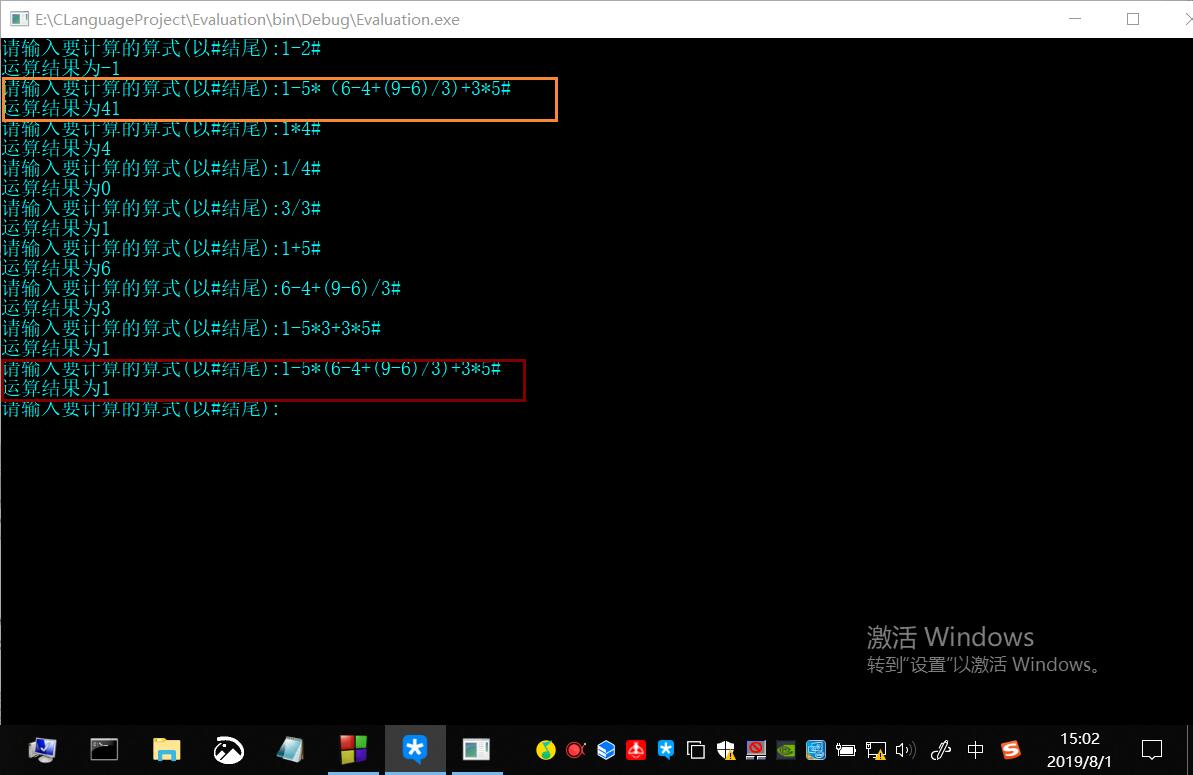展开全文四则运算 栈的应用
• 1中缀转后缀 需要建一个操作符栈op和一个字符数组expop栈存放操作符字符数组用来存放转换以后的后缀表达式首先得到用户输入的中缀表达式将其存入str数组中对str数组逐个扫描如果是数字或小数点则直接存入exp数组中当...
• 中缀转后缀 需要建一个操作符栈op和一个字符数组expop栈存放操作符字符数组用来存放转换以后的后缀表达式首先得到用户输入的中缀表达式将其存入str数组中对str数组逐个扫描如果是数字或小数点则直接存入exp数组中当...
• 然后对后缀表达式进行计算具体实现方法如下 1中缀转后缀 需要建一个操作符栈 op 和一个字符数组 expop 栈存放操作符 字符数组用来存 放转换以后的后缀表达式首先得到用户输入的中缀表达式将其存入 str 数组中 str ...
• 这里限定的表达式求值问题是:用户输入一个包含“+”、“-”、“*”、“/”、正整数和圆括号的合法数学表达式,计算该表达式的运算结果 2.输入 表达式字符串 3.输出 表达式值 4.样例输入 15 5.提示 1）运算符...
一、实验要求

1.描述

这里限定的表达式求值问题是:用户输入一个包含“+”、“-”、“*”、“/”、正整数和圆括号的合法数学表达式,计算该表达式的运算结果

2.输入

表达式字符串

3.输出

表达式值

4.样例输入

15

5.提示

1）运算符优先级关系

＋

－

×

／

(

)

#

＋

>

>

<

<

<

>

>

－

>

>

<

<

<

>

>

×

>

>

>

>

<

>

>

／

>

>

>

>

<

>

>

(

<

<

<

<

<

=

)

>

>

>

>

>

>

#

<

<

<

<

<

=

2）涉及的基本操作（函数）

函数名

功能

函数名

功能

InitStack()

初始化栈

Push()

入栈

GetTop()

获栈顶元素

Precede()

优先级比较

Pop()

出栈

Operate()

运算

3）解题思想实现算法描述

OperandType EvaluateExpression()
{ //算术表达式求值的算符优先算法。设OPTR和OPND分别为运算符栈和运算数栈，OP为运算符的集合。
InitStack(OPTR);Push(OPTR,'#');
InitStack(OPND);c=getchar();
while(c!='#'||GetTop(OPTR)!='#')
{
if(!In(c,OP))
{
Push((OPND,c);
c=getchar();
}
//不是运算符则进栈
else
switch(Precede(GetTop(OPTR), c)
{
case '<'://栈顶元素优先权低
Push(OPTR,c); c=getchar(); break;
case '=':// 脱括号并接收下一个字符
Pop(OPTR, x); c=getchar(); break;
case ‘>’://出栈，并将运算结果入栈
Pop(OPTR,theta);
Pop(OPND,b); Pop(OPND,a);
Push(OPND,Operate(a,theta,b));
}//swith
}//while
return GetTop(OPND);
}//EvaluateExpression

二、需求分析

本实验通过C语言来实现数据结构中的栈，以及通过使用栈这个数据结构完成1~9整数之间的加、减、乘、除四则混合运算的实验，并完成指定的测试操作。

从键盘输入：数字1-9、“(”左括号、“)”右括号、“+”加号、“-”减号、“*”乘号、“/”除号构成的表达式并以“#”号结尾，结果输出：形如：“结果=”+表达式的值。
本程序没有边界约束，违反输入规则的均会导致程序运行失败。
三、概要设计

1.抽象数据类型的定义

typedef struct{
SElemType *base; //在栈构造之前和销毁之后，base的初值为NULL
SElemType *top; //栈顶指针，设栈顶、栈底指针的目的是便于判断栈是否为空
int stacksize; //当前已分配的存储空间，以元素为单位.
}SqStack;//栈


2.主程序的流程图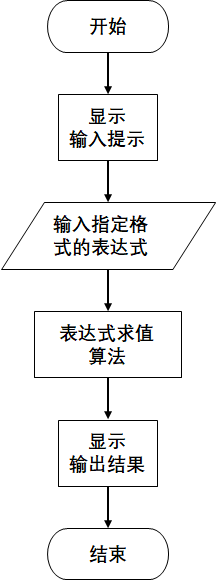3.本程序各功能模块之间均不互相调用，单独成块，由主函数一一调用。

四、详细设计

1.程序开始预编译部分如下：

//头文件
#include <stdio.h>
#include <stdlib.h>

// 函数结果状态代码
#define OVERFLOW -2
#define TRUE 1
#define FALSE 0
#define OK 1
#define ERROR 0

typedef int Status;

typedef char RElemtype;
typedef int DElemtype;

//内存空间数量级
#define STACK_INIT_SIZE 100
#define STACKINCREMENT 10

typedef struct {
RElemtype *base;
RElemtype *top;
int stacksize;
} SqStack1;//运算符栈类型定义

typedef struct {
DElemtype *base;
DElemtype *top;
int stacksize;
} SqStack2;//操作数栈类型定义


2.程序主要功能函数如下：

DElemtype EvaluateExpression();//计算表达式值
Status OPTR_InitStack(SqStack1 *S);//运算符栈的初始化
Status OPND_InitStack(SqStack2 *S);//操作数栈的初始化
RElemtype OPTR_GetTop(SqStack1 S);//取运算符栈的栈顶元素
DElemtype OPND_GetTop(SqStack2 S);//取操作数栈的栈顶元素
Status OPTR_Push(SqStack1 *S, RElemtype e);//压栈
Status OPND_Push(SqStack2 *S, DElemtype e);//压栈
RElemtype OPTR_Pop(SqStack1 *S, RElemtype *e);//出栈
DElemtype OPND_Pop(SqStack2 *S, DElemtype *e);//出栈
char Precede(char a, char b);//运算符优先级比较
DElemtype Operate(DElemtype d, char e, DElemtype f);//操作数d、f参加e运算的结果
extern int In(char c);//判断c是否是运算符

3.函数调用关系图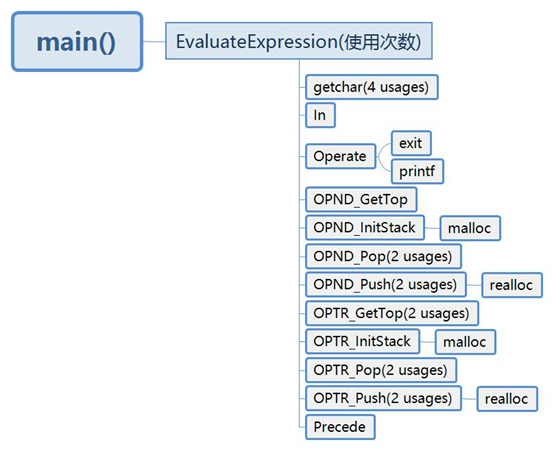五、调试分析

在EvaluateExpression()表达式计算中，默认开始初始化OPTR运算符栈和OPND运算数栈，在运算符栈的栈底要默认有一个“#”号用于结束表达式的计算和字符输入。刚开始我没加，导致程序一直需要输入字符，无法结束，之后加上，解决。
In(char c)运算符判断函数，刚开始写时忘记加入左右括号和“#”号的判断，没有对“#”号导致EvaluateExpression()函数中的while循环成死循环状态，最终使程序陷入死循环；没有判断括号，导致括号入错栈，造成表达式的计算的顺序和计算的数的位置出现问题，括号被做为数值参与到运算，同时导致运算数栈存在计算完成，元素仍有存留的现象，最终导致计算结果不正确。
如何将输出的char字符转成正确的int数值存入操作数栈中？解决办法：每次存入OPND的char字符减去字符‘0’的ASCII值从而得到正确的int数值，在存入其中。
比较两个运算符的优先级函数char Precede(char a, char b)思路解析：
运算符优先级比较表：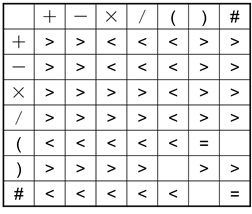将运算符之间的优先级制作成一张表格，使用二维数组存放；

    char pre[] = {
//将运算符之间的优先级制作成一张表格
{'>', '>', '<', '<', '<', '>', '>'},
{'>', '>', '<', '<', '<', '>', '>'},
{'>', '>', '>', '>', '<', '>', '>'},
{'>', '>', '>', '>', '<', '>', '>'},
{'<', '<', '<', '<', '<', '=', '0'},
{'>', '>', '>', '>', '0', '>', '>'},
{'<', '<', '<', '<', '<', '0', '='}
};


通过两个switch语句来定位需要判断符号的优先级所对应的数组的一维下标和二维下标，取出指定的元素，'>'表示a>b；'<'表示a<b；'0'表示不可能出现的比较；“(“=”)”表示左右括号相遇，括号内的运算已经完成， “#“=”#” 表达式求值完成。

switch (a) {
case '+':i = 0;break;
case '-':i = 1;break;
case '*':i = 2;break;
case '/':i = 3;break;
case '(':i = 4;break;
case ')':i = 5;break;
case '#':i = 6;break;
default:return OVERFLOW;
}
switch (b) {
case '+':j = 0;break;
case '-':j = 1;break;
case '*':j = 2;break;
case '/':j = 3;break;
case '(':j = 4;break;
case ')':j = 5;break;
case '#':j = 6;break;
default:return OVERFLOW;
}


六、测试数据与结果

1.开始界面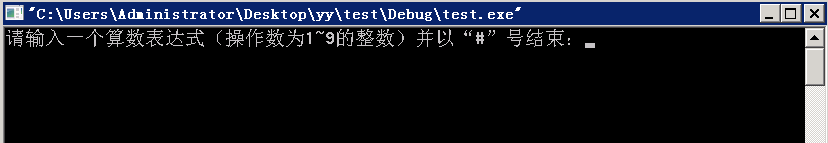2.输入 指定格式的运算符表达式（因为格式有所要求，故不做边界测试）

如：2+3x(5+6-3x2)+4/(6-4)-3x3+1=11

结果如下：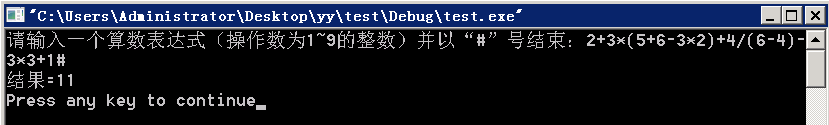3.输出结果格式：“结果=” +表达式的值

实验成功！

附录——源代码清单

fun.h

//头文件
#include <stdio.h>
#include <stdlib.h>

// 函数结果状态代码
#define OVERFLOW -2
#define TRUE 1
#define FALSE 0
#define OK 1
#define ERROR 0

typedef int Status;

typedef char RElemtype;
typedef int DElemtype;

//内存空间数量级
#define STACK_INIT_SIZE 100
#define STACKINCREMENT 10

typedef struct {
RElemtype *base;
RElemtype *top;
int stacksize;
} SqStack1;//运算符栈类型定义

typedef struct {
DElemtype *base;
DElemtype *top;
int stacksize;
} SqStack2;//操作数栈类型定义

extern DElemtype EvaluateExpression();//计算表达式值
extern Status OPTR_InitStack(SqStack1 *S);//运算符栈的初始化
extern Status OPND_InitStack(SqStack2 *S);//操作数栈的初始化
extern RElemtype OPTR_GetTop(SqStack1 S);//取运算符栈的栈顶元素
extern DElemtype OPND_GetTop(SqStack2 S);//取操作数栈的栈顶元素
extern Status OPTR_Push(SqStack1 *S, RElemtype e);//压栈
extern Status OPND_Push(SqStack2 *S, DElemtype e);//压栈
extern RElemtype OPTR_Pop(SqStack1 *S, RElemtype *e);//出栈
extern DElemtype OPND_Pop(SqStack2 *S, DElemtype *e);//出栈
extern char Precede(char a, char b);//运算符优先级比较
extern DElemtype Operate(DElemtype d, char e, DElemtype f);//操作数d、f参加e运算的结果
extern int In(char c);//判断c是否是运算符


main.c

#include "fun.h"
/**
* 默认栈底有一个结束符‘#’
* 操作符忘记判断#号 (号 )号  死了多次
* 操作数ascii值转int再运算 死了两次
*/
Status OPTR_InitStack(SqStack1 *S) {//运算符栈的初始化
//构造一个空栈S
S->base = (RElemtype *) malloc(STACK_INIT_SIZE * sizeof(RElemtype));
if (!S->base) return (OVERFLOW); //存储分配失败
S->top = S->base;
S->stacksize = STACK_INIT_SIZE;
return OK;
}

Status OPND_InitStack(SqStack2 *S) {//操作数栈的初始化
//构造一个空栈S
S->base = (DElemtype *) malloc(STACK_INIT_SIZE * sizeof(DElemtype));
if (!S->base) return (OVERFLOW); //存储分配失败
S->top = S->base;
S->stacksize = STACK_INIT_SIZE;
return OK;
}

RElemtype OPTR_GetTop(SqStack1 S) {//取运算符栈的栈顶元素
if (S.top == S.base) return ERROR;//若栈不空，则用e返回S的栈顶元素，并返回OK；否则返回ERROR
return *(S.top - 1); //栈顶指针不变
}

DElemtype OPND_GetTop(SqStack2 S) {//取操作数栈的栈顶元素
if (S.top == S.base) return ERROR;//若栈不空，则用e返回S的栈顶元素，并返回OK；否则返回ERROR
return *(S.top - 1); //栈顶指针不变
}

Status OPTR_Push(SqStack1 *S, RElemtype e) {//压栈
//插入元素e为新的栈顶元素
if (S->top - S->base >= S->stacksize) {//栈满，追加存储空间
S->base = (RElemtype *) realloc(S->base, (S->stacksize + STACKINCREMENT) * sizeof(RElemtype));
if (!S->base) return (OVERFLOW);
S->top = S->base + S->stacksize;
S->stacksize += STACKINCREMENT;
}
*S->top++ = e;
return OK;
}

Status OPND_Push(SqStack2 *S, DElemtype e) {//压栈
//插入元素e为新的栈顶元素
if (S->top - S->base >= S->stacksize) {//栈满，追加存储空间
S->base = (DElemtype *) realloc(S->base, (S->stacksize + STACKINCREMENT) * sizeof(DElemtype));
if (!S->base) return (OVERFLOW);
S->top = S->base + S->stacksize;
S->stacksize += STACKINCREMENT;
}
*S->top++ = e;
return OK;

}

RElemtype OPTR_Pop(SqStack1 *S, RElemtype *e) {//出栈
//若栈不空，则删除S的栈顶元素，用e返回其值，并返回OK；否则返回ERROR
if (S->top == S->base) return ERROR; //栈空
*e = *--S->top;
return *e;
}

DElemtype OPND_Pop(SqStack2 *S, DElemtype *e) {//出栈
//若栈不空，则删除S的栈顶元素，用e返回其值，并返回OK；否则返回ERROR
if (S->top == S->base) return ERROR; //栈空
*e = *--S->top;
return *e;
}

char Precede(char a, char b) {
/**
* 比较两个运算符的优先级；
* a，b中存放待比较的运算符；
* '>'表示a>b；
* '0'表示不可能出现的比较.
*/
int i, j;
char pre[] = {
//将运算符之间的优先级制作成一张表格
{'>', '>', '<', '<', '<', '>', '>'},
{'>', '>', '<', '<', '<', '>', '>'},
{'>', '>', '>', '>', '<', '>', '>'},
{'>', '>', '>', '>', '<', '>', '>'},
{'<', '<', '<', '<', '<', '=', '0'},
{'>', '>', '>', '>', '0', '>', '>'},
{'<', '<', '<', '<', '<', '0', '='}
};
switch (a) {
case '+':
i = 0;
break;
case '-':
i = 1;
break;
case '*':
i = 2;
break;
case '/':
i = 3;
break;
case '(':
i = 4;
break;
case ')':
i = 5;
break;
case '#':
i = 6;
break;
default:
return OVERFLOW;
}
switch (b) {
case '+':
j = 0;
break;
case '-':
j = 1;
break;
case '*':
j = 2;
break;
case '/':
j = 3;
break;
case '(':
j = 4;
break;
case ')':
j = 5;
break;
case '#':
j = 6;
break;
default:
return OVERFLOW;
}
return pre[i][j];
}

DElemtype Operate(DElemtype d, char e, DElemtype f) {//操作数d、f参加e运算的结果
DElemtype i = d, j = f, result;
switch (e) {
case '+':
result = i + j;
break;
case '-':
result = i - j;
break;
case '*':
result = i * j;
break;
case '/':
result = i / j;
break;
default://可能不会被使用
printf("出现非法运算符，程序终止！");
exit(0);
}
return result;
}

int In(char c) {//判断c是否是运算符
return c == '+' ||
c == '-' ||
c == '*' ||
c == '/' ||
c == '(' ||
c == ')' ||
c == '#' ?
TRUE : FALSE;
}

DElemtype EvaluateExpression() {//计算表达式值
/**
* 算术表达式求值的算符优先算法；
*设OPTR和OPND分别为运算符栈和运算数栈；
*/
SqStack1 OPTR;
SqStack2 OPND;
DElemtype a, b;
char flag;
char c, x, theta;
OPTR_InitStack(&OPTR);
OPTR_Push(&OPTR, '#');//默认栈底有一个结束符‘#’
OPND_InitStack(&OPND);
c = getchar();
while (c != '#' || OPTR_GetTop(OPTR) != '#') {
if (In(c) != TRUE) {//不是运算符则进操作数栈
OPND_Push(&OPND, c - '0');

c = getchar();
} else {
flag = Precede(OPTR_GetTop(OPTR), c);
switch (flag) {
case '<'://栈顶元素优先权低
OPTR_Push(&OPTR, c);
c = getchar();
break;
case '='://脱括号并接收下一字符
OPTR_Pop(&OPTR, &x);
c = getchar();
break;
case '>'://退栈并将运算结果入栈
OPTR_Pop(&OPTR, &theta);
OPND_Pop(&OPND, &b);
OPND_Pop(&OPND, &a);
OPND_Push(&OPND, Operate(a, theta, b));
break;
}//swith
}
}//while
return OPND_GetTop(OPND);
}//EvaluateExpression

int main() {
DElemtype c;
printf("请输入一个算数表达式（操作数为1~9的整数）并以“#”号结束：");
c = EvaluateExpression();
printf("结果=%d\n", c);
}



展开全文数据结构 程序设计
• 数据结构（C语言）栈的应用——编写一个四则运算计算器（输入数学公式得出计算结果） 文章目录数据结构（C语言）栈的应用——编写一个四则运算计算器（输入数学公式得出计算结果）前言一、需要用到的知识点1.栈的...
数据结构（C语言）栈的应用——编写一个四则运算计算器（输入数学公式得出计算结果）

文章目录数据结构（C语言）栈的应用——编写一个四则运算计算器（输入数学公式得出计算结果）前言一、需要用到的知识点1.栈的特点2.要先明白怎么让计算机认得你输入的数学表达式2.1解决方案——将表达式转为后缀表达式二、有了理论知识后我们开搞1.准备工作2.大致的实现过程3.个函数实现的详细步骤3.1： int LeftPri(char op);      //左运算符op的优先级和 int RightPri(char op);     //右运算符op的优先级3.2：   bool InOp(char ch)        //判断ch是否为运算符3.3：int ConpareCode(char op1, char op2);  //比较op1与op2的优先级3.4：void Transform(char* exp, char postExp[]); //将exp（用户输入的表达式）转变成栈中元素，通过栈的特性将表达式转换为后缀表达式并存入postExp[]中3.5：float Compute(char * postExp) //进行后缀表达式运算，返回结果4. 最终效果总结附录（全部的源代码）

前言
栈真的很神奇当你第一次学到它的时候会觉得就这？一个结构体中定义了一个数组，和一个整型变量指向数组，实在是想不出它有什么作用，存储数据用数组和链表不香吗？直到发现它在计算器中的应用才发现栈是永远的神！

一、需要用到的知识点

1.栈的特点
定义一个栈
struct
{
char data[MaxSize];  //储存数据的数组,MaxSize是栈储存最大容量
int top;             //int 类型的“指针”
} op;                    //用top来对data中数组的操作

2.要先明白怎么让计算机认得你输入的数学表达式
比如你在键盘上输入(7+6×3)/(2+3)这个全部可以用字符串数组来保存，将运算符与数字分离出也不太难，正真要费点脑子的是搞清楚各种符号的优先级我们人当然知道在上述公式中6×3优先于7+6而括号中的（7+6×3）与（2+3）优先于/ 所以怎么让计算机理解这个是本程序的最大难点。
2.1解决方案——将表达式转为后缀表达式
同样上述例子将其转换为7#6#3#×+2#3+/ 用#分隔数字将运算符号后置，保存在一个栈中，设计一个算法只运算前两位将结果又储存进栈 （这个栈就暂定义为op），就可以实现运算符号优先级运算了。
这里参考“数据结构教程”：二、有了理论知识后我们开搞

1.准备工作
这里定义了两个特殊的结构体数组，左结构体数组表示栈op的顶元素（op.data[op.top]）中的运算字符的优先级（简单来说就是用先储存进来的运算符与后储存进来的运算符做比较）右结构体数组表示exp（后进来的运算符）
#include <iostream>
#include <stdio.h>
#include <stdlib.h>

#define MaxSize 30

struct
{				//设定运算符优先级
char ch;    //运算符
int pri;    //优先级
}leftPri[] = { {'=', 0}, {'(', 1}, {'*', 5}, {'/', 5}, {'+', 3}, {'-', 3}, {')', 6} },
rightPri[] = { {'=', 0}, {'(', 6}, {'*', 4}, {'/', 4}, {'+', 2}, {'-', 2}, {')', 1} };
//rightPri[]与leftPri[]表示运算符相同时优先级会比低的数学意义是“先左后右”

2.大致的实现过程
int LeftPri(char op);      //左运算符op的优先级
int RightPri(char op);     //右运算符op的优先级
bool InOp(char ch);        //判断ch是否为运算符
int ConpareCode(char op1, char op2);  //比较op1与op2的优先级
void Transform(char* exp, char postExp[]); //将exp（用户输入的表达式）转变成栈中元素，通过栈的特性将表达式转换为后缀表达式并存入postExp[]中
float Compute(char* postExp);           //进行后缀表达式运算，返回结果

int main()
{
char exp;
printf("计算器：\n\n");
printf("请输入数学计算公式：");
scanf("%s", exp);
char postExp[MaxSize];
Transform(exp, postExp);
printf("答案：%g\n\n", Compute(postExp));

return 0;
}


3.个函数实现的详细步骤
3.1： int LeftPri(char op);      //左运算符op的优先级和 int RightPri(char op);     //右运算符op的优先级
总的来说：就是输入一个运算符返回与之对应的优先级数
int LeftPri(char op)      //左运算符op的优先级
{
for(int i = 0; i < 7; i++)  //遍历leftPri[]数组，找到与之对应的优先级并返回
if (leftPri[i].ch == op)
return leftPri[i].pri;

}

int RightPri(char op)     //右运算符op的优先级
{
int i;
for (int i = 0; i < 7; i++)
if (rightPri[i].ch == op)
return rightPri[i].pri;
}

3.2：   bool InOp(char ch)        //判断ch是否为运算符
一个小组件函数
bool InOp(char ch)        //判断ch是否为运算符
{
if (ch == '(' || ch == ')' || ch == '+' || ch == '-' || ch == '*' || ch == '/')
return true;
else
return false;
}

3.3：int ConpareCode(char op1, char op2);  //比较op1与op2的优先级
一个比较小组件函数
int ConpareCode(char op1, char op2)
{
if (LeftPri(op1) == RightPri(op2))
return 0;
else if (LeftPri(op1) < RightPri(op2))
return -1;
else
return 1;
}

3.4：void Transform(char* exp, char postExp[]); //将exp（用户输入的表达式）转变成栈中元素，通过栈的特性将表达式转换为后缀表达式并存入postExp[]中
这个函数可以算是这个程序最复杂的，一定要认真看才能看的懂
void Transform(char* exp, char postExp[])
{
struct    //建立一个临时的栈，
{
char data[MaxSize];
int top;
} op;
int i = 0;
int flag = 0;
op.top = -1; //令其指向空
op.top++;
op.data[op.top] = '=';   //top = 0，将栈顶存入“=”做为结束的标语
while (*exp != '\0')
{
if (!InOp(*exp))   //将数字与运算符号分开
{
while (*exp >= '0' && *exp <= '9')
{
postExp[i++] = *exp;
exp++;
}
postExp[i++] = '#'; //在每个数字后加一个“#”将连续的数字分开
}
else
{
switch (ConpareCode(op.data[op.top], *exp)) //注意每次都跟栈顶比
{
case -1:           //*exp中的运算符优先级高将它入栈
op.top++;     //top = 1
op.data[op.top] = *exp;
exp++;        //继续遍历
break;
case 0:           //只有“=”和“（”才会出现这种情况，
op.top--; //top = 0   //如果先前碰到了就将原有的运算符退栈，可以认为将（+）中的+输入到poseExp中
exp++;
break;
case 1:
postExp[i++] = op.data[op.top];  // 如果栈顶优先级高就直接输入进postExp
op.top--;
break;
}
}

}
while (op.data[op.top] != '=') //减到0之后就退出，
{

postExp[i++] = op.data[op.top];
op.top--;  //top的值越大，优先级就越高！
}
postExp[i] = '\0'; //表字符串结束
}

3.5：float Compute(char * postExp) //进行后缀表达式运算，返回结果
float Compute(char * postExp)
{
struct
{
float data[MaxSize];   //定义一个载体栈
int top;
} ser;

float a, b, c, d;
ser.top = -1;
while (*postExp != '\0')
{
switch (*postExp)   //进行判断运算字符
{
case '+':
a = ser.data[ser.top];
ser.top--;
b = ser.data[ser.top];
ser.top--;
c = a + b;
ser.top++;
ser.data[ser.top] = c;  //将结果存入原来 b 值的位置
break;
case '-':
a = ser.data[ser.top];
ser.top--;
b = ser.data[ser.top];
ser.top--;
c = b - a;
ser.top++;
ser.data[ser.top] = c;
break;
case '*':
a = ser.data[ser.top];
ser.top--;
b = ser.data[ser.top];
ser.top--;
c = b * a;
ser.top++;
ser.data[ser.top] = c;
break;
case '/':
a = ser.data[ser.top];
ser.top--;
b = ser.data[ser.top];
ser.top--;
if (a!= 0)   //除数不能为零
{
c = b / a;
ser.top++;
ser.data[ser.top] = c;
}
else
{
printf("\n\t格式错误");
exit(0);
}

break;
default:
d = 0;
while (*postExp >= '0' && *postExp <= '9')
{
d = 10 * d + *postExp - '0'; //将数字剥离出来
postExp++;               //两个postExp++恰好跳过了‘#’符号
}
ser.top++;
ser.data[ser.top] = d;

}
postExp++;  //循环继续
}
return(ser.data[ser.top]);  返回结果
}


4. 最终效果
输入公式输出结果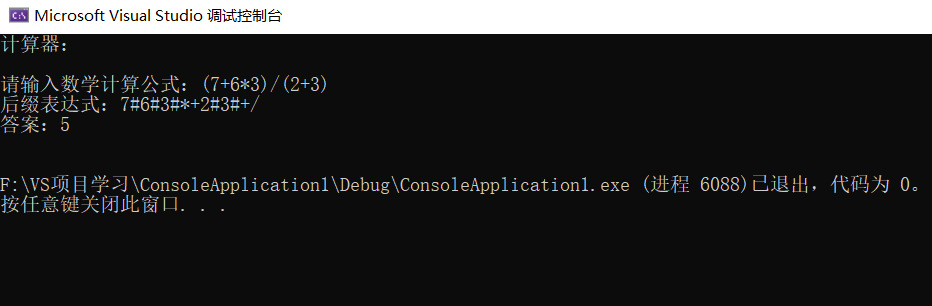总结

程序的基本步骤为：输入表达式—>转换为后缀表达式—>计算—>输出。为实现看似简单的数学基本运算C语言的代码行数竟干到了200行，而其中函数几个功能对于新手来说比较硬核，但当你搞明白了之后你就会发现支持这个计算器底层运行规律的不是代码而是数学
万物基于C语言（×）
万物基于数学（√）

附录（全部的源代码）



#include <iostream>
#include <stdio.h>
#include <stdlib.h>

#define MaxSize 30

struct          //设定运算符优先级
{				//运算符
char ch;    //优先级
int pri;
}leftPri[] = { {'=', 0}, {'(', 1}, {'*', 5}, {'/', 5}, {'+', 3}, {'-', 3}, {')', 6} },
rightPri[] = { {'=', 0}, {'(', 6}, {'*', 4}, {'/', 4}, {'+', 2}, {'-', 2}, {')', 1} };

int LeftPri(char op);      //左运算符op的优先级
int RightPri(char op);     //右运算符op的优先级
bool InOp(char ch);        //判断ch是否为运算符
int ConpareCode(char op1, char op2);  //比较op1与op2的优先级
void Transform(char* exp, char postExp[]); //将exp（用户输入的表达式）转变成栈中元素，
float Compute(char* postExp);           //进行后缀表达式运算，返回结果

int main()
{
char exp;
printf("计算器：\n\n");
printf("请输入数学计算公式：");
scanf("%s", exp);
char postExp[MaxSize];
Transform(exp, postExp);
printf("答案：%g\n\n", Compute(postExp));

return 0;
}

int LeftPri(char op)      //左运算符op的优先级
{
for(int i = 0; i < 7; i++)
if (leftPri[i].ch == op)
return leftPri[i].pri;

}

int RightPri(char op)     //右运算符op的优先级
{
int i;
for (int i = 0; i < 7; i++)
if (rightPri[i].ch == op)
return rightPri[i].pri;
}

bool InOp(char ch)        //判断ch是否为运算符
{
if (ch == '(' || ch == ')' || ch == '+' || ch == '-' || ch == '*' || ch == '/')
return true;
else
return false;
}

int ConpareCode(char op1, char op2)
{
if (LeftPri(op1) == RightPri(op2))
return 0;
else if (LeftPri(op1) < RightPri(op2))
return -1;
else
return 1;
}

void Transform(char* exp, char postExp[])
{
struct
{
char data[MaxSize];
int top;
} op;
int i = 0;
int flag = 0;
op.top = -1;
op.top++;
op.data[op.top] = '=';   //top = 0
while (*exp != '\0')
{
if (!InOp(*exp))
{
while (*exp >= '0' && *exp <= '9')
{
postExp[i++] = *exp;
exp++;
}
postExp[i++] = '#';
}
else
{
switch (ConpareCode(op.data[op.top], *exp)) //top = 0即op.data[op.top] = '='
{
case -1:
op.top++;     //top = 1
op.data[op.top] = *exp;
exp++;
break;
case 0:
op.top--; //top = 0
exp++;
break;
case 1:
postExp[i++] = op.data[op.top];
op.top--;
break;
}
}

}
while (op.data[op.top] != '=') //减到0之后就退出，
{

postExp[i++] = op.data[op.top];
op.top--;  //top的值越大，优先级就越高！
}
postExp[i] = '\0';
}

float Compute(char * postExp)
{
struct
{
float data[MaxSize];
int top;
} ser;

float a, b, c, d;
ser.top = -1;
while (*postExp != '\0')
{
switch (*postExp)
{
case '+':
a = ser.data[ser.top];
ser.top--;
b = ser.data[ser.top];
ser.top--;
c = a + b;
ser.top++;
ser.data[ser.top] = c;
break;
case '-':
a = ser.data[ser.top];
ser.top--;
b = ser.data[ser.top];
ser.top--;
c = b - a;
ser.top++;
ser.data[ser.top] = c;
break;
case '*':
a = ser.data[ser.top];
ser.top--;
b = ser.data[ser.top];
ser.top--;
c = b * a;
ser.top++;
ser.data[ser.top] = c;
break;
case '/':
a = ser.data[ser.top];
ser.top--;
b = ser.data[ser.top];
ser.top--;
if (a!= 0)
{
c = b / a;
ser.top++;
ser.data[ser.top] = c;
}
else
{
printf("\n\t格式错误");
exit(0);
}

break;
default:
d = 0;
while (*postExp >= '0' && *postExp <= '9')
{
d = 10 * d + *postExp - '0';
postExp++;               //两个postExp++恰好跳过了‘#’符号
}
ser.top++;
ser.data[ser.top] = d;

}
postExp++;
}
return(ser.data[ser.top]);
}




展开全文数据结构 算法 抽象代数
• 你不用成为数学天才，只要使用算术运算符按正确顺序把数值排列起来组成一个数学算术表达式(expression)，C语言就会完成具体的数学计算。一个算术表达式包含了一个或多个运算符以及常量、变量或数值。C语言中，当给...
数学运算是计算机最基本的功能，C语言是由各种算术运算符(operator)来完成数学运算的。你不用成为数学天才，只要使用算术运算符按正确顺序把数值排列起来组成一个数学算术表达式(expression)，C语言就会完成具体的数学计算。一个算术表达式包含了一个或多个运算符以及常量、变量或数值。C语言中，当给变量赋值时，经常在赋值运算符=右侧使用算术表达式，比如：Score = Maths + English + Science;               //计算三门课总成绩Average = (Maths + English + Science) / 3;       //计算平均成绩C 程序会计算出结果并将其存储在变量 Score 和 Average 中。表 1 列出了 C 语言中常用的 5 种算术运算符。表 1：C语言中常用的算术运算符说明运算符意义说明+加法–减法如果减去一个负数，则-左右必须加空格*乘法/除法两个整数相除结果是整数(小数部分被截取)；两个数中有一个是浮点数，其结果就是浮点数%取模求整数除法的余数，其正负取决于被除数这五种运算符的运算优先级为*=/=%>+=-，即 *、/、% 具有相同的优先级，它们的级别大于 + 和 -，+ 和 – 具有相同的优先级；优先级相同时按从左向右的顺序运算。使用括号可以打破上述优先级规则，括号具有最高的优先级。在 C语言中的加、减、乘与通常数学运算中的定义完全相同，几乎可以用于所有数据类型；而除法运算在 C语言中较为特殊，详述如下。1) 除法运算C语言中使用/对整型数据进行除运算时，结果的小数部分将被截掉，其被看成是“整除运算”；但若除数或被除数有一个是带小数位的实数，则被看成是“实数除法”，结果中的小数位将进行四舍五入处理。例如：int Average = 8 / 3;               //运行后变量 Average 的值为 2float Average = 8 / 3;             //运行后变量 Average 的值为 2.000000float Average = 8 / 3.0;           //运行后变量 Average 的值为 2.666667float Average = 8.0 / 3;           //运行后变量 Average 的值为 2.666667float Average = 8.0 / 3.0;         //运行后变量 Average 的值为 2.6666672) 求模运算用%求除法余数的运算在编程中称为求模。求模运算只能用于整型数据。例如：int a = 8 % 3;                //运行后变量 a 的值为 2，即 8 除以 3 的余数 23) 字符型数据的算术运算在 C语言中，字符型的数据也可以参加算术运算。前面讲过，字符在计算机中也是以数字的形式存在的，每一个字符都对应于一个数字(见 ASCII 标准字符代码表)，因而字符参加算术运算实际上就是对应字符的十进制字符代码参加运算。例如：int Su = 'A' + 'B' + 20;     //运行后变量 Su 的值为 151在“ASCII 标准字符代码表”中，字符 ‘A’ 的十进制字符代码是 65，字符 ‘B’ 的十进制字符代码是 66，因而上述语句的计算结果相当于以下语句：int Su = 65 + 66 + 20;
展开全文• 一般普通计算器都是计算两个数相乘，除，加，减，即时计算出答案，而并不能实现输入一个简单数学算式并进行计算得出结果。对于很多人来说，没有合适的方法计算一个算式，那么我们其实也可以通过栈来实现计算算式。 ...C 计算
• 一、程序通过编译，并实现两个命题的各种逻辑运算 二、任意输入字符串P和Q逻辑表达式的合法性检查 三、利用真值表方法验证他们的等价性
• C程序实验报告电子数学C程序实验报告电子数学实验五 C语言程序初步一、实验目的熟悉Turbo C与Visual C++程序运行环境。掌握如何编辑、编译、链接和运行一个C程序。掌握Ｃ语言程序的书写格式和Ｃ语言程序的结构。了解...
• 高中数学大家都学过数列，其中一个重要的概念就是数列的通项，可以代表数列中每一项的一个表达式。 今天我们的问题就跟通项有关系，说，给你一个数列的通项和数列中的前几项，希望你能求出它的第n项。 通项表达式...
• 被控系统的数学模型是描述系统内在物理量之间关系的数学表达式。系统的数学模型体现了输入量、输出量之间的内在关系，将输入量与输出量通过物理关系连接起来。认清被控系统的数学模型，是设计控制系统的基础。从另外...控制算法
• 程序可以直接输入数学表达式，不需要任何转换，就可以直接输出数学四则运算的结果。并且操作简单、界面清晰、灵活、实用、方便等要求出发，它所能完成的主要计算功能有以下几个方面：加法，减法，乘法，除法，求幂，...
• （1）输入不含变量的数学表达式的中缀形式，可以接受的操作符包括+、-、*、/、%； （2）若表达式正确，则输出表达式的结果；若表达式非法，则输出错误信息； （3）同时输出堆栈的状态变化过程； （4）有合理的...c++
• 公式为C 顺序结构练习2 输入三角形的三边长求三角形面积 若输入的三边长abc能构成三角形 数学知识已知求三角形面积的公式为 其中s=(a+b+c)/2 注意在C语言程序中如何表达这些数学表达式 由于要调用数学函数库中的函数...
• C语言课件(顺序结构程序设计)解析顺序结构程序设计 顺序结构程序设计 基本结构 语句 输入输出 字符输入/输出函数 格式输入/输出函数 数学库函数 基本结构 顺序结构 选择结构 循环结构 语句 语句是构成程序的最小成分...
• 输入数学表达式 输入为数字 和“+ - * / ^(平方) r（根号）s（sin) c(cos) t(tan) l(ln)以及括号" 2、思路 （1）识别小数和大于10的数，和运算符同时存入double类型的数组 （2）改写为后缀表达式 （3）用后缀表达式...stack
• sin函数是数学函数，其头文件为math.h文件，因此在程序的主函数前用include命令包含了math.h。scanf和printf是标准输入输出函数，其头文件为stdio.h，在主函数前也用include命令包含了stdio.h文件。 　需要说明的是...
• 在近两个月的学习中，由为什么学习C语言，到后来的逐渐接触C语言，慢慢地去认识... 在随后的顺序，选择，循环语句的学习中，我慢慢感觉到了C语言对我们生活的影响，例如在数学的研究和大数据的计算方面起到了非常大...
• 数学表达式与库函数-银行存款本息计算 【问题描述】 设银行定期存款的年利率rate为2.25%，已知存款期为n年，存款本金为capital元，试编程计算并输出n年后的本利之和deposit。 编程提示：编程提示：乘方计算用...
• 计算数学公式/数学表达式** 重点：数学公式，Format表达式（小数点） %6.2f 表示一共6位数，其中两位是小数,不足六位前面补空格，小数点不算一位 1)关于圆 2）从键盘输入小于1000的正数，要求输出平方根，如果...
• FSM（finite state machine），它是一种协议，是一个数学概念，用于有限数量的子程序（状态）的变化，每个子程序进行一些...C语言中的状态机表达式通常使用函数指针数组的方式。 void （*state[MAX_STATES] ) ( )； ex
• 速算24扑克游戏的规则是由系统发出4张扑克牌，用户利用扑克牌的数字及运算符号“+”，“-”，“*”，“/”，及“（”和“）”组成一个计算表达式，从键盘上输入该计算表达式，系统运行后得出计算结果，如果结果等于...
• 有两个人AB在数学考试中获得87和76的成绩请输入AB两人的代号及成绩输出成绩 ;任务2 总分及平均分的计算;三相关知识(一) 算术运算与算术表达式(一) 算术运算与算术表达式 (二) 赋值运算和赋值表达式;例1-12阅读下
• 　最近FJ为他的奶牛们开设了数学分析课，FJ知道若要学好这门课，必须有一个好的三角函数基本功。所以他准备和奶牛们做一个“Sine之舞”的游戏，寓教于乐，提高奶牛们的计算能力。 　不妨设 　An=sin(1–sin(2+sin(3...
• 第二章 C 语言程序设计基础 学习目标 掌握 C 语言的数据类型常量和变量的使用 掌握常用的运算符和表达式的使用 掌握库函数 scanf)printf) putchar) getchar) 的使用 能够编写程序解决一些简单的数学计算问题 学习...
• 目录第1章　C语言概述第2章　C语言基本概念第3章　格式化的输入/输出第4章　表达式第5章　选择语句第6章　循环第7章　基本类型　第8章　数组第9章　函数第10章　程序结构第11章　指针第12章　指针和数组第13章　...
• A.7 表达式 A.8 说明 A.9 语句 A.10 外部说明 A.11 作用域与连接 A.12 预处理 A.13 语法 附录B 标准库 B.1 输入与输出：<stdio.h> B.2 字符类测试：<ctype.h> B.3 字符串函数：<string.h> B.4 数学函数：......

# c语言输入数学表达式c语言 订阅